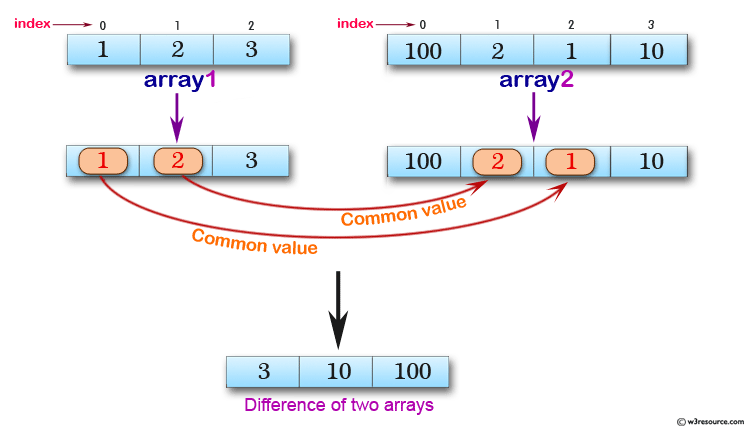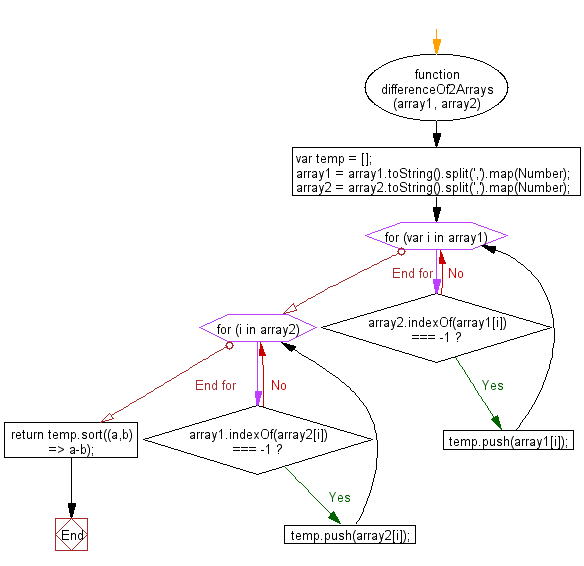﻿ JavaScript array: Find the difference of two arrays - w3resource# JavaScript: Find the difference of two arrays

## JavaScript Array: Exercise-23 with Solution

Write a JavaScript function to find the difference of two arrays.

Test Data:
console.log(difference([1, 2, 3], [100, 2, 1, 10]));
["3", "10", "100"]
console.log(difference([1, 2, 3, 4, 5], [1, , [3, []],[5,6]]));
["6"]
console.log(difference([1, 2, 3], [100, 2, 1, 10]));
["3", "10", "100"]

Pictorial Presentation:Sample Solution:

HTML Code:

``````<!DOCTYPE html>
<html>
<meta charset="utf-8">
<title>JS, difference between two arrays</title>
<body>
</body>
</html>
```
```

JavaScript Code:

``````function differenceOf2Arrays (array1, array2) {
var temp = [];
array1 = array1.toString().split(',').map(Number);
array2 = array2.toString().split(',').map(Number);

for (var i in array1) {
if(array2.indexOf(array1[i]) === -1) temp.push(array1[i]);
}
for(i in array2) {
if(array1.indexOf(array2[i]) === -1) temp.push(array2[i]);
}
return temp.sort((a,b) => a-b);
}

console.log(differenceOf2Arrays([1, 2, 3], [100, 2, 1, 10]));
console.log(differenceOf2Arrays([1, 2, 3, 4, 5], [1, , [3, []],[5,6]]));
```
```

Sample Output:

```[3,10,100]

```

Flowchart:ES6 Version:

``````function differenceOf2Arrays (array1, array2) {
const temp = [];
array1 = array1.toString().split(',').map(Number);
array2 = array2.toString().split(',').map(Number);

for (var i in array1) {
if(!array2.includes(array1[i])) temp.push(array1[i]);
}
for(i in array2) {
if(!array1.includes(array2[i])) temp.push(array2[i]);
}
return temp.sort((a,b) => a-b);
}

console.log(differenceOf2Arrays([1, 2, 3], [100, 2, 1, 10]));
console.log(differenceOf2Arrays([1, 2, 3, 4, 5], [1, , [3, []],[5,6]]));
``````

Live Demo:

See the Pen JavaScript - Find the difference of two arrays - array-ex- 23 by w3resource (@w3resource) on CodePen.

Improve this sample solution and post your code through Disqus

What is the difficulty level of this exercise?

Test your Programming skills with w3resource's quiz.

﻿

## JavaScript: Tips of the Day

var keyword

```var num = 8;
var num = 10;

console.log(num);
```

With the var keyword, you can declare multiple variables with the same name. The variable will then hold the latest value.
You cannot do this with let or const since they're block-scoped.

Ref: https://bit.ly/323Y0P6